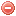# Description

The Orieux index was developed by Orieux (1974) in order to predict fire danger in southeastern France (Mediterranean climate). This simple daily index is based on the principle that fire danger mostly depends on wind and soil moisture, and requires wind speed, temperature and precipitation as input variables.

The level of fire danger for the coming day is calculated in two steps. First, the estimated soil water reserve is determined by considering the daily balance between rainfall and evapotranspiration in a fictive reservoir which is saturated when water reaches 150 [mm]. Then, the estimated water reserve is combined to the next day's forecasted wind speed (Orieux 1974, Chandler et al. 1983, Sol 1990).

As the estimated soil water reserve is aimed to reflect the water stress of vegetation, the Orieux index is suitable only in summer (between mid-June until the first rainfalls at the end of summer; Sol 1990).

# Formula

Orieux (1974) set the maximum water reserve of the soil $$r_{max}$$ to 150 [mm], and assumed that the soil water reserve r decreases exponentially as a function of the cumulated daily values of evapotranspiration:

$r=r_{max}\cdot{e^ {-\frac{\sum PET}{r_{max}}}}$

where $$r$$ is the estimated water reserve in the soil [mm], $$r_{max}$$ the maximum water reserve of the soil, i.e. 150 [mm], and $$PET$$ the daily potential evapotranspiration [mm] according to the Thornthwaite (1948) equation.

Soil water content, i.e. the balance between water removal (evapotranspiration) and supply (rainfall) is determined as follows: If no rainfall occurs, the water reserve of the soil $$r$$ decreases according to the above equation. If rainfall occurs, the water reserve increases, and then decreases again as previously explained when rainfall stops. When the water reserve reaches 150 [mm], the soil is then satured and water trickles.

In the original publication it is not clear which procedure has to be applied to calculate the index. This could in fact be calculated simply according to the balance or adapting the above equation to work in case of rainfall. Here we list the options that, to our knowledge, could work.

The daily water balance $$r_t$$ could be calculated as follow:

$r_t= min \Big[r_{max}, \ r_{t-1} + P_t - \dfrac{r_{t-1}}{r_{max}}\cdot PET_t\Big]$

where $$P$$ is daily rainfall [mm].

Otherwise we could use following procedure:

$r_t=r_{max}\cdot{e^ {-\frac{PETcum_t}{r_{max}}}}$

with

$PETcum_t= \begin{cases} PETcum_{t-1} + PET_t, & \mbox{if }P_t=0 \\ max \Bigg[0, -r_{max} \cdot ln \Bigg(\dfrac{ r_{t-1} + P_t - \frac{r_{t-1}}{r_{max}}\cdot PET_t }{r_{max}}\Bigg)\Bigg], & \mbox{if }P_t>0 \\ \end{cases}$

thus, in case of rain, recalculating the cumulative of $$PET$$ for the corresponding value of $$r$$.

The Orieux index is supposed to be calculated on a daily basis.

The index computation should be started when the water reserve $$r$$ is saturated (150 [mm]), as e.g. after strong rainfall. The first index value $$r$$ is then set to 150.

# Index interpretation

Fire danger classes are determined according to the following table:

Estimated water reserve [mm] Wind speed [km/h]
< 20 20-40 > 40
100-150 0 0 0
50-100 1 1 2
30-50 1 2 3
< 30 1 2 3

where 0 corresponds to a low, 1 to an usual, 2 to a high, and 3 to a very high fire danger.

Original publication:
Orieux (1974)

Other publications:
Thornthwaite (1948)
Chandler et al. (1983)
Sol (1990)

### Bibliography SearchNo results!

No results were found. Are you sure you searched for a tag?

### Symbols

 Variable Description Unit $$T$$ air temperature °C $$T_{dew}$$ dew point temperature °C $$H$$ air humidity % $$P$$ rainfall mm $$U$$ windspeed m/s $$w$$ days since last rain (or rain above threshold) d $$rr$$ days with consecutive rain d $$\Delta t$$ time increment d $$\Delta{e}$$ vapor pressure deficit kPa $$e_s$$ saturation vapor pressure kPa $$e_a$$ actual vapor pressure kPa $$p_{atm}$$ atmospheric pressure kPa $$PET$$ potential evapotranspiration mm/d $$r$$ soil water reserve mm $$r_s$$ surface water reserve mm $$EMC$$ equilibrium moisture content % $$DF$$ drought factor - $$N$$ daylight hours hr $$D$$ weighted 24-hr average moisture condition hr $$\omega$$ sunset hour angle rad $$\delta$$ solar declination rad $$\varphi$$ latitude rad $$Cc$$ cloud cover Okta $$J$$ day of the year (1..365/366) - $$I$$ heat index - $$R_n$$ net radiation MJ⋅m-2⋅d-1 $$R_a$$ daily extraterrestrial radiation MJ⋅m-2⋅d-1 $$R_s$$ solar radiation MJ⋅m-2⋅d-1 $$R_{so}$$ clear-sky solar radiation MJ⋅m-2⋅d-1 $$R_{ns}$$ net shortwave radiation MJ⋅m-2⋅d-1 $$R_{nl}$$ net longwave radiation MJ⋅m-2⋅d-1 $$\lambda$$ latent heat of vaporization MJ/kg $$z$$ elevation m a.s.l. $$d_r$$ inverse relative distance Earth-Sun - $$\alpha$$ albedo or canopy reflection coefficient - $$\Delta$$ slope of the saturation vapor pressure curve kPa/°C $$Cc$$ cloud cover eights $$ROS$$ rate of spread m/h $$RSF$$ rate of spread factor - $$WF$$ wind factor - $$WRF$$ water reserve factor - $$FH$$ false relative humidity - $$FAF$$ fuel availability factor - $$PC$$ phenological coefficient -

 Suffix Description $$-$$ mean / daily value $$_{max}$$ maximum value $$_{min}$$ minimum value $$_{12}$$ value at 12:00 $$_{13}$$ value at 13:00 $$_{15}$$ value at 15:00 $$_{m}$$ montly value $$_{y}$$ yearly value $$_{f/a}$$ value at fuel-atmosphere interface $$_{dur}$$ duration $$_{soil}$$ value at soil level

 Constant Description $$e$$ Euler's number $$\gamma$$ psychrometric constant $$G_{SC}$$ solar constant $$\sigma$$ Stefan-Bolzmann constant